# (2 + 1)维Hirota-Satsuma-Ito方程的呼吸波与不同非线性波之间的态转换State Transformations between the Breather Wave and Different Nonlinear Waves for the (2 + 1)-Dimensional Hirota-Satsuma-Ito Equation

DOI: 10.12677/AAM.2021.104149, PDF, HTML, XML, 下载: 26  浏览: 81

Abstract: In this paper, the (2 + 1)-dimensional Hirota-Satsuma-Ito equation is studied based on the Hirota bilinear method. Under certain circumstances, the breather wave can be transformed into other types of nonlinear waves, including W-type, M-type, oscillating-W-type, oscillating-M-type and quasi-periodic-type waves, and the dynamic characteristics of these nonlinear waves are analyzed. Based on the characteristic line analysis, the conversion condition between the breather wave and other nonlinear waves is obtained. The results enrich the dynamic characteristics of the (2 + 1)-dimensional nonlinear waves.

1. 引言

${u}_{x}\left(3\int {u}_{t}\text{d}x+\gamma \right)+3u{u}_{t}+{u}_{xxt}+\int {u}_{yt}\text{d}x=0,$ (1)

2. 呼吸波解和态转换条件

$u=2{\left[\mathrm{ln}f\left(x,y,t\right)\right]}_{xx},$ (2)

$\left({D}_{t}{D}_{x}^{3}+\gamma {D}_{x}^{2}+{D}_{t}{D}_{y}\right)f\cdot f=0,$ (3)

${D}_{x}^{p}{D}_{y}^{q}f\cdot g={\left(\frac{\partial }{\partial x}-\frac{\partial }{\partial {x}^{\prime }}\right)}^{p}{\left(\frac{\partial }{\partial y}-\frac{\partial }{\partial {y}^{\prime }}\right)}^{q}f\left(x,y\right)\cdot g\left({x}^{\prime },{y}^{\prime }\right)|{}_{\left(x,y\right)=\left({x}^{\prime },{y}^{\prime }\right)}.$ (4)

${f}_{2}=1+{\text{e}}^{{\theta }_{1}}+{\text{e}}^{{\theta }_{2}}+{\text{e}}^{{\theta }_{1}+{\theta }_{2}}{d}_{12}.$ (5)

${\theta }_{i}={k}_{i}x+{l}_{i}y+{\omega }_{i}t+{\delta }_{i},\left(i=1,2\right)$，其中

${\omega }_{i}=-\frac{\gamma {k}_{i}^{2}}{{k}_{i}^{3}+{l}_{i}},\left(i=1,2\right),{d}_{12}=-\frac{\left({\omega }_{1}-{\omega }_{2}\right){\left({k}_{1}-{k}_{2}\right)}^{3}+\left({\omega }_{1}-{\omega }_{2}\right)\left({l}_{1}-{l}_{2}\right)+\gamma {\left({k}_{1}-{k}_{2}\right)}^{2}}{\left({\omega }_{1}+{\omega }_{2}\right){\left({k}_{1}+{k}_{2}\right)}^{3}+\left({\omega }_{1}+{\omega }_{2}\right)\left({l}_{1}+{l}_{2}\right)+\gamma {\left({k}_{1}+{k}_{2}\right)}^{2}},$ (6)

${k}_{i},{l}_{i},{\delta }_{i}\left(i=1,2\right)$ 是一些自由参量，我们对这些参量进行复数化，即：

${k}_{1}={a}_{1}+i{b}_{1}={k}_{2}^{*},{l}_{1}={c}_{1}+i{d}_{1}={l}_{2}^{*},{\delta }_{1}=\mathrm{ln}\frac{{\lambda }_{1}}{2}+{\beta }_{1}+i{\eta }_{1}={\delta }_{2}^{*},$ (7)

*代表共轭， ${a}_{1},{b}_{1},{c}_{1},{d}_{1},{\lambda }_{1}\left(>0\right),{\beta }_{1},{\eta }_{1}$ 都是实常数。将式(6)和(7)代入到式(5)中，可以得到

${f}_{2}~{\lambda }_{1}\mathrm{cos}\left({\Lambda }_{1}\right)+2\sqrt{{\lambda }_{2}}\mathrm{cosh}\left({\xi }_{1}+\frac{1}{2}\mathrm{ln}{\lambda }_{2}\right),$ (8)

${\xi }_{1}={a}_{1}x+{c}_{1}y+{\omega }_{1R}t+{\beta }_{1},{\Lambda }_{1}={b}_{1}x+{d}_{1}y+{\omega }_{1I}t+{\eta }_{1},{\lambda }_{2}=\frac{1}{4}{\lambda }_{1}^{2}{d}_{12},$ (9)

$\begin{array}{l}{\omega }_{1R}=-\frac{\gamma \left({a}_{1}^{5}+2{a}_{1}^{3}{b}_{1}^{2}+{a}_{1}^{2}{c}_{1}-{b}_{1}^{2}{c}_{1}+{a}_{1}\left({b}_{1}^{4}+2{b}_{1}{d}_{1}\right)\right)}{{\left({a}_{1}^{3}-3{a}_{1}{b}_{1}^{2}+{c}_{1}\right)}^{2}+{\left(3{a}_{1}^{2}{b}_{1}-{b}_{1}^{3}+{d}_{1}\right)}^{2}},\\ {\omega }_{1I}=\frac{\gamma \left({a}_{1}^{4}{b}_{1}+{b}_{1}^{5}-2{a}_{1}{b}_{1}{c}_{1}-{b}_{1}^{2}{d}_{1}+{a}_{1}^{2}\left(2{b}_{1}^{3}+{d}_{1}\right)\right)}{{\left({a}_{1}^{3}-3{a}_{1}{b}_{1}^{2}+{c}_{1}\right)}^{2}+{\left(3{a}_{1}^{2}{b}_{1}-{b}_{1}^{3}+{d}_{1}\right)}^{2}}.\end{array}$ (10)

$\begin{array}{c}u\left[x,y,t\right]=\frac{8{a}_{1}{b}_{1}{\lambda }_{1}\sqrt{{\lambda }_{2}}\mathrm{sin}\left({\Lambda }_{1}\right)\mathrm{sinh}\left({\xi }_{1}+\frac{1}{2}\mathrm{ln}{\lambda }_{2}\right)+8{a}_{1}^{2}{\lambda }_{2}-2{b}_{1}^{2}{\lambda }_{1}^{2}}{{\left[{\lambda }_{1}\mathrm{cos}\left({\Lambda }_{1}\right)+2\sqrt{{\lambda }_{2}}\mathrm{cosh}\left({\xi }_{1}+\frac{1}{2}\mathrm{ln}{\lambda }_{2}\right)\right]}^{2}}\\ \text{\hspace{0.17em}}+\frac{4\left({a}_{1}^{2}-{b}_{1}^{2}\right){\lambda }_{1}\sqrt{{\lambda }_{2}}\mathrm{cos}\left({\Lambda }_{1}\right)\mathrm{cosh}\left({\xi }_{1}+\frac{1}{2}\mathrm{ln}{\lambda }_{2}\right)}{{\left[{\lambda }_{1}\mathrm{cos}\left({\Lambda }_{1}\right)+2\sqrt{{\lambda }_{2}}\mathrm{cosh}\left({\xi }_{1}+\frac{1}{2}\mathrm{ln}{\lambda }_{2}\right)\right]}^{2}}.\end{array}$ (11)

$\begin{array}{l}{L}_{1}={a}_{1}x+{c}_{1}y+{\omega }_{1R}t+{\beta }_{1}+\frac{1}{2}\mathrm{ln}{\lambda }_{2}=0,\\ {L}_{2}={b}_{1}x+{d}_{1}y+{\omega }_{1I}t+{\eta }_{1}=0.\end{array}$ (12)

${a}_{1}{d}_{1}-{b}_{1}{c}_{1}=0,$ (13)

3. 转换波的类型

3.1. W型、M型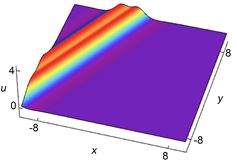(a)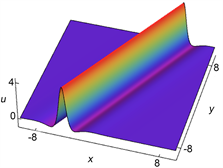(b)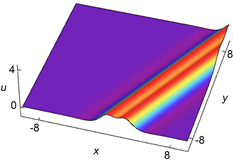(c)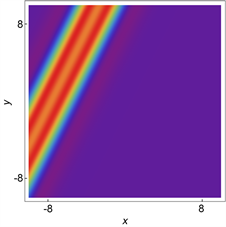(d)(e)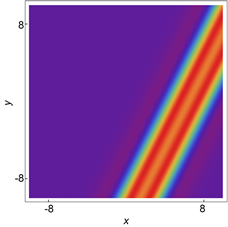(f)

Figure 1. Converted waves at different times: (a) t = −19; (b) t = 4.35; (c) t = 21.06; (d), (e) and (f) are the corresponding density maps respectively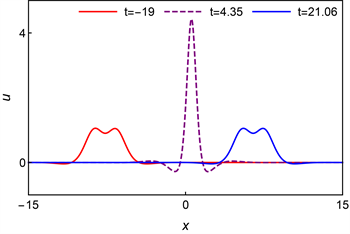Figure 2. Sectional view of three moments

3.2. 振荡W型、振荡M型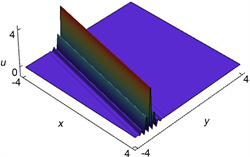(a)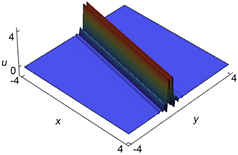(b)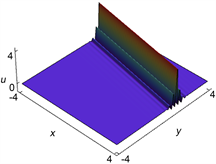(c)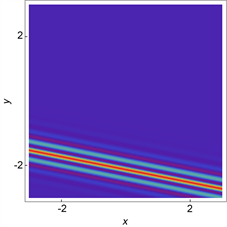(d)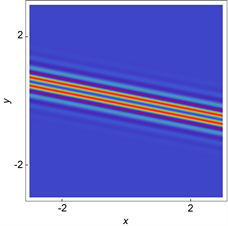(e)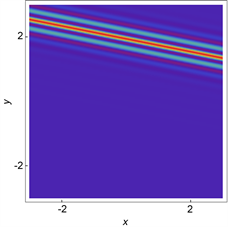(f)

Figure 3. Converted waves at different times: (a) t = −58.6; (b) t = 20.95; (c) t = 92.9; (d), (e) and (f) are the corresponding density maps respectively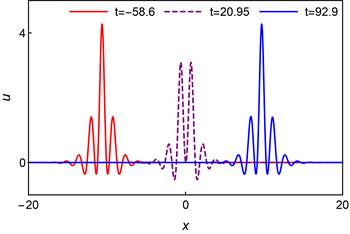Figure 4. Sectional view of three moments

3.3. 准周期型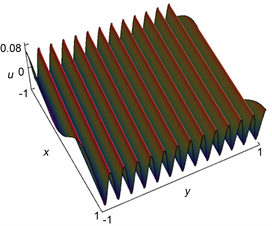(a)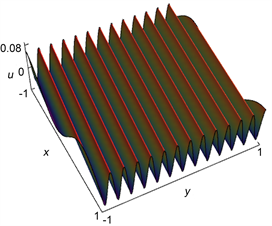(b)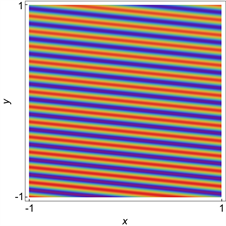(c)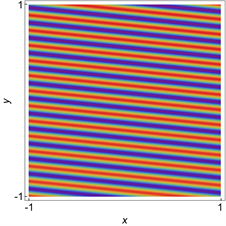(d)

Figure 5. Converted waves at different times: (a) t = 37; (b) t = 45; (c) t = 92.9; (c) and (d) are the corresponding density maps respectively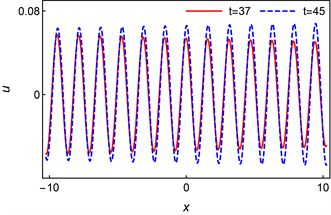Figure 6. Sectional view of two moments

4. 结论

  Solli, D.R., Ropers, C., Koonath, P. and Jalali, B. (2007) Optical Rogue Waves. Nature, 450, 1054-1057. https://doi.org/10.1038/nature06402  Bludov, Y.V., Konotop, V.V. and Akhmediev, N. (2009) Matter Rogue Waves. Physical Review A, 80, Article ID: 033610. https://doi.org/10.1103/PhysRevA.80.033610  Chabchoub, A., Hoffmann, N.P. and Akhmediev, N. (2011) Rogue Wave Observation in a Water Wave Tank. Physical Review Letters, 106, Article ID: 204502. https://doi.org/10.1103/PhysRevLett.106.204502  Kibler, B., Fatome, J., Finot, C., Millot, G., Dias, F., Genty, G., Akhmediev, N. and Dudley, J.M. (2010) The Peregrine Soliton in Nonlinear Fibre Optics. Nature Physics, 6, 790-795. https://doi.org/10.1038/nphys1740  Bailung, H., Sharma, S.K. and Nakamura, Y. (2011) Observation of Peregrine Solitons in a Multi-Component Plasma with Negative Ions. Physical Review Letters, 107, Article ID: 255005. https://doi.org/10.1103/PhysRevLett.107.255005  Dai, C.Q., Wang, Y.Y. and Zhang, J.F. (2020) Managements of Scalar and Vector Rogue Waves in a Partially Nonlocal Nonlinear Medium with Linear and Harmonic Potentials. Nonlinear Dynamics, 102, 379-391. https://doi.org/10.1007/s11071-020-05949-0  Akhmediev, N., Soto-Crespo, J.M. and Ankiewicz, A. (2009) Extreme Waves That Appear from Nowhere: On the Nature of Rogue Waves. Physics Letters A, 373, 2137-2145. https://doi.org/10.1016/j.physleta.2009.04.023  Agrawal, G.P. (2006) Nonlinear Fiber Optics. 4th Edition, Academic Press, San Diego. https://doi.org/10.1016/B978-012369516-1/50011-X  Ma, W.X. (2015) Lump Solutions to the Kadomtsev-Petviashvili Equation. Physics Letters A, 379, 1975-1978. https://doi.org/10.1016/j.physleta.2015.06.061  Singh, N. and Stepanyants, Y. (2016) Obliquely Propagating Skew KP Lumps. Wave Motion, 64, 92-102. https://doi.org/10.1016/j.wavemoti.2016.03.005  Zhou, Y., Manukure, S. and Ma, W.X. (2019) Lump and Lump-Soliton Solutions to the Hirota-Satsuma-Ito Equation. Communications in Nonlinear Science and Numerical Simulation, 68, 56-62. https://doi.org/10.1016/j.cnsns.2018.07.038  Zhou, Y. and Manukure, S. (2019) Complexiton Solutions to the Hirota-Satsuma-Ito Equation. Mathematical Methods in the Applied Sciences, 42, 2344-2351. https://doi.org/10.1002/mma.5512  Liu, Y.Q., Wen, X.Y. and Wang, D.S. (2019) The N-Soliton Solution and Localized Wave Interaction Solutions of the (2+1)-Dimensional Generalized Hirota-Satsuma-Ito Equation. Computers & Mathematics with Applications, 77, 947-966. https://doi.org/10.1016/j.camwa.2018.10.035  Liu, J.G., Zhu, W.H. and Li, Z. (2020) Multi-Wave, Breather Wave, and Interaction Solutions of the Hirota-Satsuma-Ito Equation. European Physical Journal plus, 135, 20. https://doi.org/10.1140/epjp/s13360-019-00049-4  Hirota, R. (2004) The Direct Method in Soliton Theory. Cambridge University Press, Cambridge. https://doi.org/10.1017/CBO9780511543043